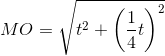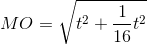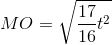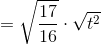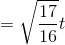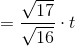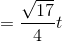## Example Questions

### Example Question #3 : Quadrilaterals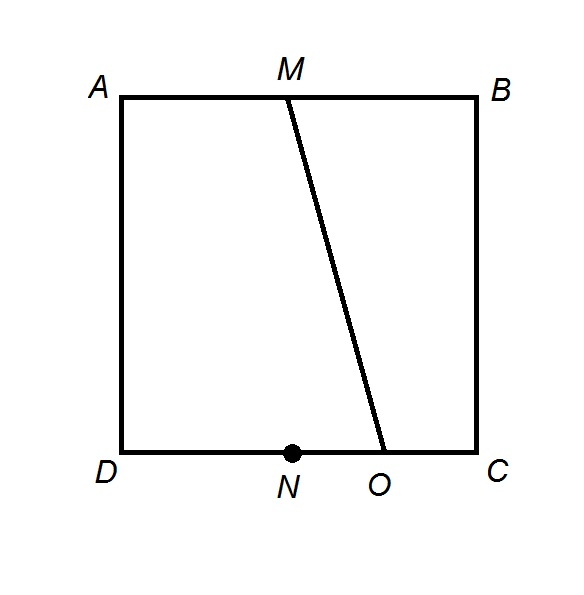The above figure shows Squareis the midpoint of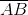is the midpoint of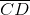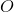is the midpoint of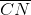. Construct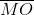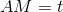. Which of the following expresses the length ofin terms of?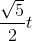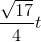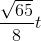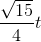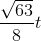Explanation:

Construct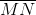as shown in the diagram below: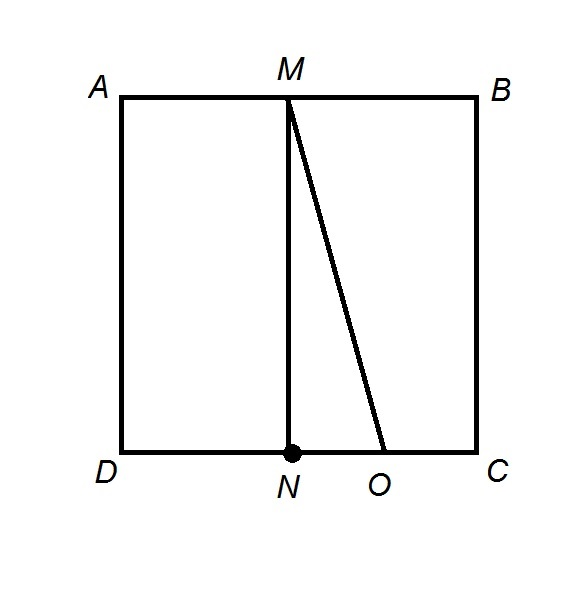Quadrilateral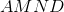is a rectangle, so opposite sides are congruent. Therefore,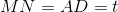.

Sinceis the midpoint of,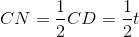Sinceis the midpoint of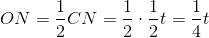.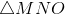is a right triangle, so, by the Pythagorean Theorem,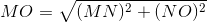Substituting: